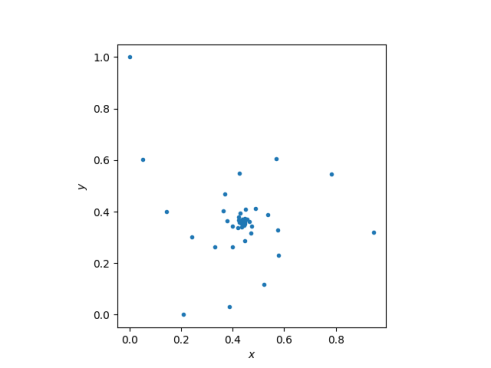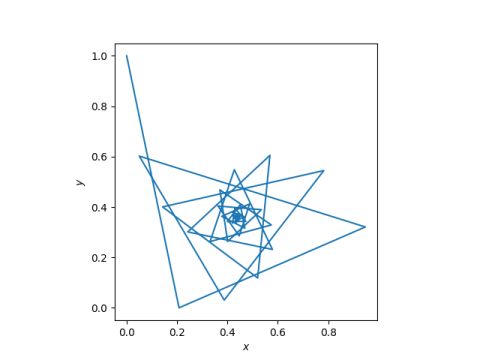# i^i^i …

This post plots the sequenceThat is, we define a sequence by z1 = i and for k > 1,I ran across this in , but the sequence goes back as far as Euler.

Here’s a plot of the points:This plot has three spiral arms, as if there are three sequences converging to the middle. Here’s a plot where we connect the dots so we can see how consecutive points rotate between the three arms.Here’s the Python code that produced the first plot.

```    import numpy as np
import matplotlib.pyplot as plt

N = 100
z = np.empty(N, dtype=complex)
z = 1j
for k in range(1, N):
z[k] = 1j**z[k-1]

fig, ax = plt.subplots()
ax.plot(z.real, z.imag, '.')
ax.set_aspect(1)
ax.set_xlabel("\$x\$")
ax.set_ylabel("\$y\$")
plt.savefig("temp.png")
```

To make the second plot, change the last argument to `ax.plot` from a period to a hyphen.

## Hyperexponentiation and tetration

The sequence in this post is hyperexponentiation of order two with base i. That is,in Donald Knuth’s up arrow notation. Using the `hyperexp` function from here, we could have generated our sequence as follows.

`    z = [hyperexp(1j, 2, n) for n in range(1, 100)]`

We use `1j` because that’s how Python represents the imaginary unit.

This sequence is also referred to as tetration. This mysterious name was coined by Reuben Goodstein by using the Greek root “tetra” for four, meaning that it is a 4th level of iteration.

In Goodstein’s scheme, the 1st level of iteration is to repeatedly add 1 to a base b. Adding 1 n times to b yields b + n. The 2nd level of iteration is to repeatedly add b to itself, yielding nb. The 3rd level of iteration is to multiply b by itself n times, yielding bn.

For k ≥ 3, Goodstein’s iteration of level k corresponds to k-2 up arrows in Knuth’s notation, hyperexponentialtion of level k – 2.

***

 Greg Parker. Complex Power Iterations. The Mathematical Gazette, Vol. 81, No. 492 (Nov., 1997), pp. 431-434

## One thought on “i^i^i …”

1. Bob Lyons

Interesting. Thanks.

I found two relevant OEIS entries:

Decimal expansion of real part of the infinite power tower of i. – https://oeis.org/A077589

Decimal expansion of imaginary part of the infinite power tower of i. – https://oeis.org/A077590